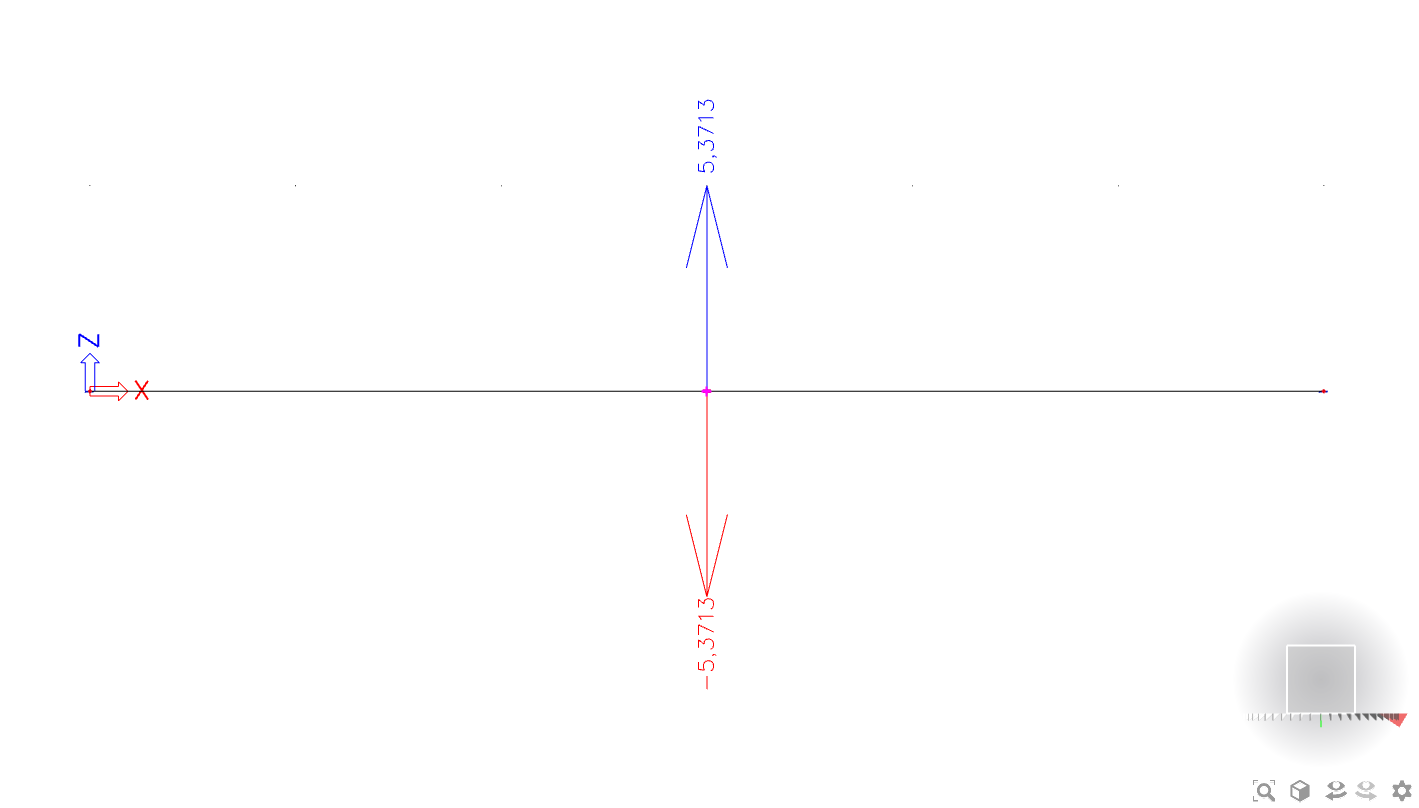# Is it possible to obtain the response of the structure as a function of the applied frequency?

When defining a dynamic harmonic load in SCIA Engineer, the frequency (and damping) is determined. The load will be entered just like a static load. If you want to define that same load with multiple frequencies, you will have to create multiple load cases in which you enter each frequency.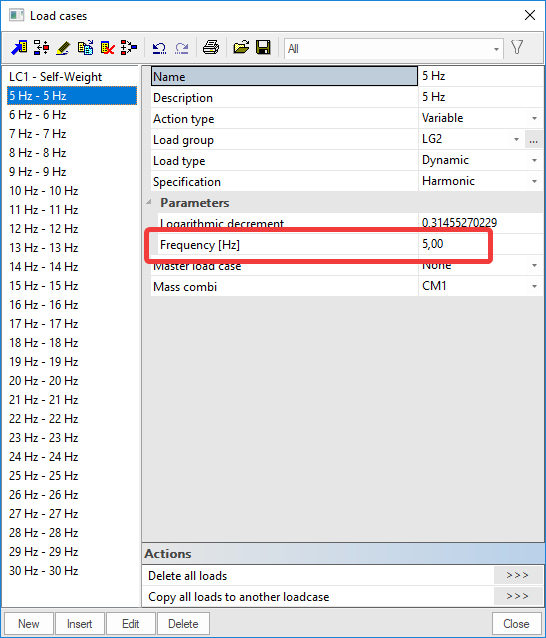Tip: By copying load cases, the contents are also copied. Therefore, the static load itself does not need to be defined again. Only the frequency has to be changed.

The load cases can then be grouped in a class.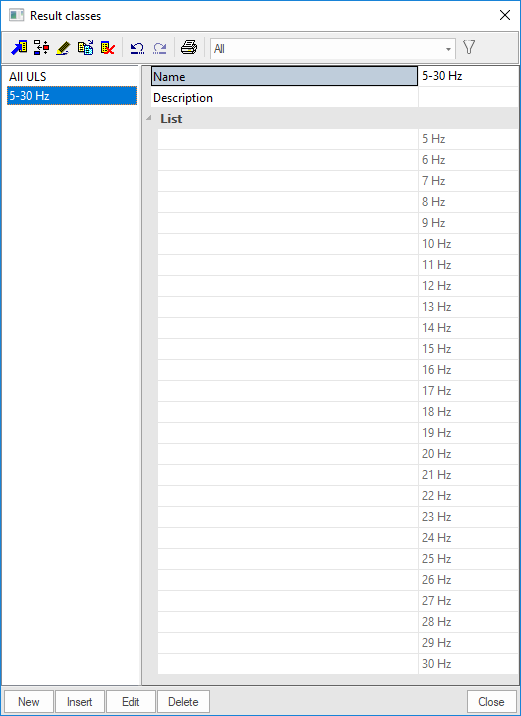After performing a linear calculation, the results can be obtained.

The results can now be requested:

• By load case, belonging to a particular frequency
• By class, the enveloping result of all the load cases

However, if you are interested in the displacement of a certain node, you can always obtain this value per load case, i.e per frequency. To do this, go to Results > Displacements of nodes. In the properties set the class containing all the frequencies along with the wanted value and set ‘Text output’ to ‘Graph’: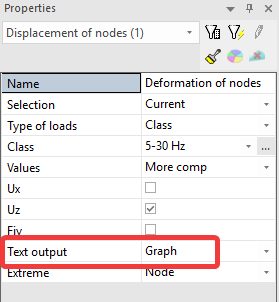When asking for the preview, the load cases (= frequencies) are plotted on the horizontal axis and the displacement is plotted on the vertical axis. This is illustrated in the following figure: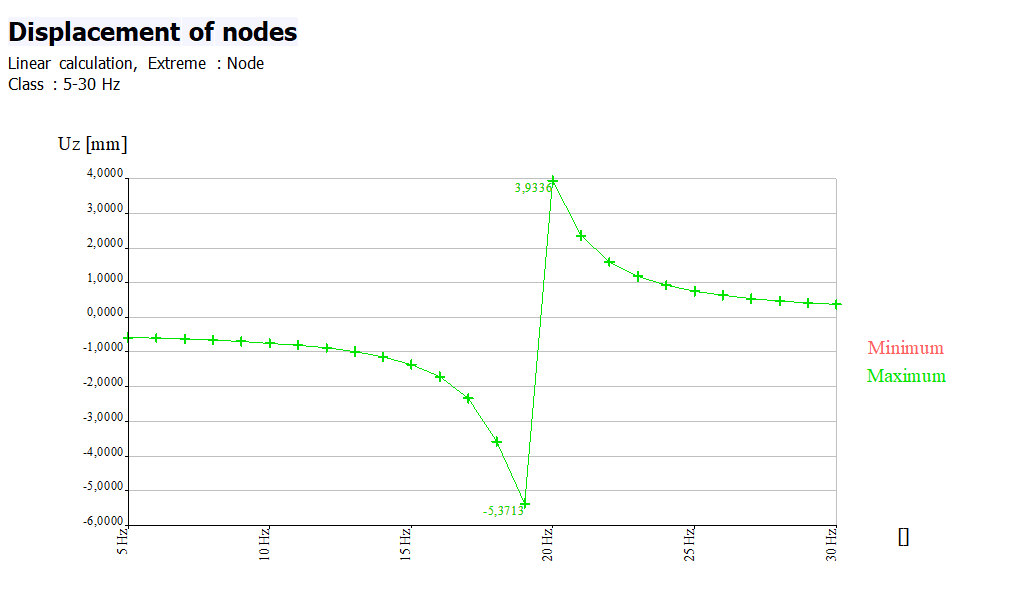In this case, the maximum displacement of the node is 5,3713mm for a frequency of 19Hz. Note that displacements of harmonic loads are always in the positive and negative direction. For frequency 19Hz this means the following: